0
410

# Puzzles Questions for SSC- CPO Set-2 PDF

Download SSC CPO Puzzles Questions with answers set-2 PDF based on previous papers very useful for SSC CPO exams. Very important Puzzles Questions for SSC exams.

Question 1: The heights of three towers are in the ratio 5 : 6 : 7. If a spider takes 15 minutes to climb the smallest tower, how much time will it take to climb the highest one?

a) 15 minutes

b) 18 minutes

c) 21 minutes

d) 54 minutes

Question 2: Six friends A, B, C, D, E and F are sitting in a circle. C is to the left of D, F is between A and E, and E is between F and D. Who is to the left of F?

a) A

b) C

c) D

d) E

Question 3: Given below are the jumbled letters of a word and their corresponding numbers. Select the combination of numbers that makes a meaningful word.C N A S P H I
1 2 3 4 5 6 7

a) 5742613

b) 2375416

c) 4572316

d) 7234516

Question 4: If BROAD means 19812, CLOCK means

a) 68262

b) 68622

c) 26826

d) 37836

Question 5: TAP is SZO, then FREEZE is

a) EQDFYG

b) EQDDYD

c) ESDFYF

d) GQFDYF

Question 6: If 876 =12, 864 = 81, 895 = 22, what is 824 = ?

a) 14

b) 64

c) 48

d) 41

Question 7: If + stands for ∗; – stands for #; × stands for @ and ÷ stands for %, then which of the following statements is correct?

a) 256% 16 @ 5 # 28 = 52

b) 256 # 16 % 5 # 28 = 120

c) 256 @ 5 % 16 ∗ 28 = 408

d) 256 # 16 @ 5 % 28 = 80

Question 8: Choose the appropriate combination of signs (in place of the three *) to solve the equation.
(16 + 18) * (21-11) * 32 * 8

a) – × =

b) – = –

c) + = –

d) ÷ – =

Question 9: Identify the number at the bottom when the top is 5 in a dice.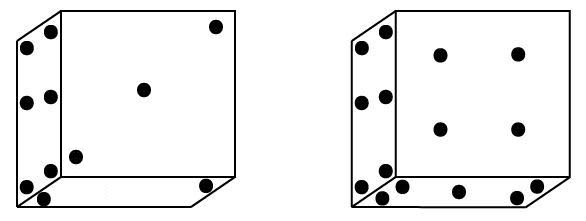a) 2

b) 3

c) 4

d) 6

Question 10: Identify the answer figure from which the pieces given in the question figure have been cut.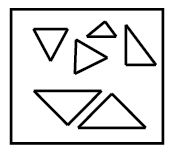a)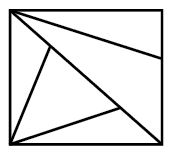b)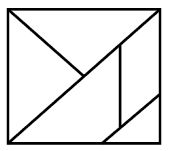c)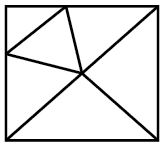d)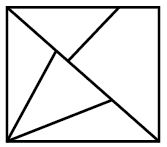Question 11: Which of the following figures will be formed when the figure given in the question is folded?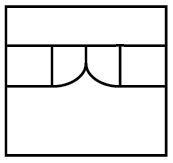a)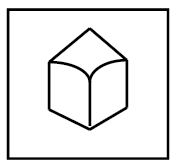b)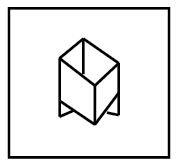c)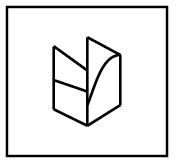d)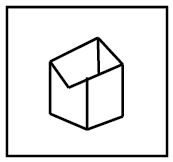Question 12: In the following diagram, the square represents college students, the,triangle represents artists, the parallelogram represents singers. Which region best represents college students who are artists as well as singers?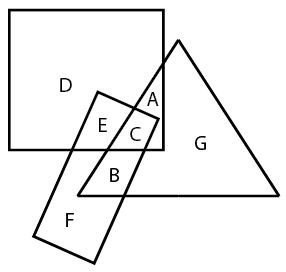a) G

b) C

c) E

d) B

Question 13: The diagram represents the number of students studying different subjects. What is the number of students who study either History or Biology ?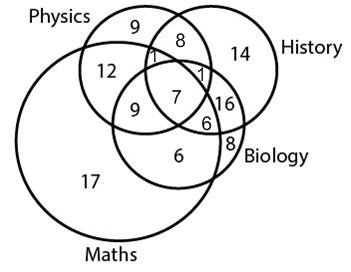a) 4

b) 16

c) 30

d) 26

Question 14: The diagram given below shows number of students who got distinction in three subjects out of 500 students. What is the percentage of students who got distinction in all subjects?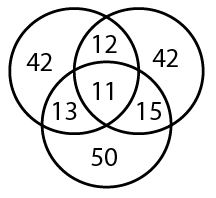a) 10.2

b) 8

c) 10

d) 2.2

Instructions

In the following questions, which answer figure will complete the pattern in the question figure?

Question 15: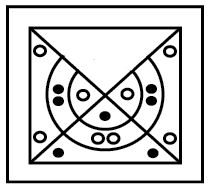a)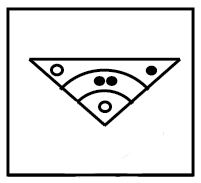b)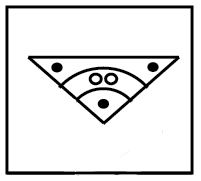c)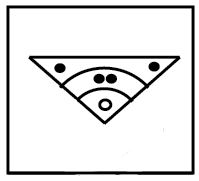d)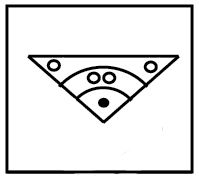We know that distance=Speed $\times$ Time.
If speed is constant than,
$\frac{Distance}{Time}$=constant.
Let height of smallest tower be ‘$5x$’.
$\therefore$ height of largest tower will be ‘$7x$’.
Let time taken by spider to climb largest time be ‘t’ minutes.
$\frac{5x}{15}=\frac{7x}{t}$
$t=21$
So, time taken by spider to climb largest tower will be 21 minutes.
Hence, Option C is correct.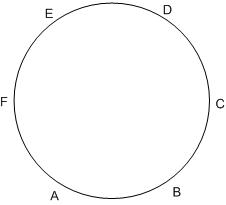Clearly, E is to the left of F.
Hence, Option D is correct.

Considering Options,
Option A 5742613=PISNHCA’.
Option B 2375416= NAIPSCH’.
Option C 4572316= ‘SPINACH’.
Option D 7234516= ‘INASPCH’.
Clearly, Only Option C i.e. SPINACH makes sense.
All other options are incorrect.
Hence, Option C is correct

Arrange letters from A to Z and assign them numbers from 1 to 26 respectively.
Each letters position is divided by 2 and take least integer value greater than the number.
E.g. {2/2}=1,{1/2}=1.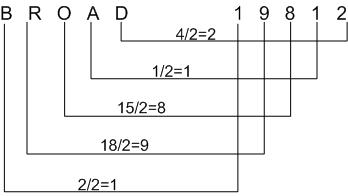Similarly,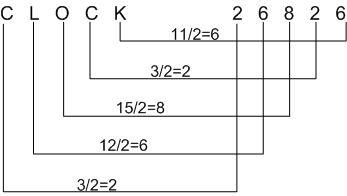Hence, Option C is correct.

Arrange Alphabet from A to Z, then we found a relation between TAP and SZO as,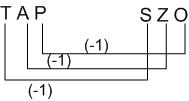Similarly,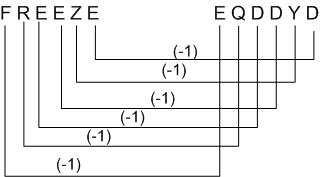Hence, Option B is the correct answer.

876-8+7+6=21=Reverse of 12.
864-8+6+4=18=Reverse of 81.
895-8+9+5=22=Reverse of 22.
Hence,824=8+2+4=14=Reverse of 41
Therefore, Option D is correct.

According to sign convention as given in question,
A-$256 \div{16} \times{5}-28=52$.
A-$16\times{5}-28=52$.
A-$80-28=52$.
A-$52=52$.
Hence, Option A is correct.

According to question,
B-$(16 + 18) -(21-11) = 32 – 8$.
B-$34-12=24$.
B-$24=24$.
Hence, Option B is correct.

From the both positions of dice, it is clear that-
No. 6 has 2,3,4,5 as its adjacent faces.
Hence, 1 is opposite to 6.
Rotating the first dice along face containing No. 6, we get Second position of dice.
Hence, 4 is opposite to 3 and 2 is opposite to 5.
Hence, When 5 is at the top, 2 is at the bottom.
Therefore, Option A is correct.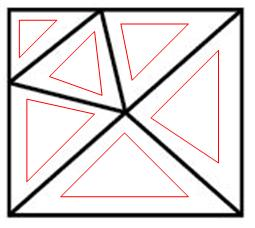From above figure, We can obtain parts as-Hence, Option C is correct.

After folding the paper, we get-Hence, Option A is correct.From the figure the college students who are artists as well as singers are those which are common to all the three figures.
Only C represents such a condition.
Hence, Option B is correct.Hence, No. of students who study history and biology=16+7+6+1.
=30.
Hence, Option C is correct.Total no. of students in the class = 500
No. of students who got distinction in all three subjects=11.
Percentage of students who got distinction in all three subjects=$\frac{11}{500}\times{100}$.
=2.2%
Hence,Option D is the correct answer.